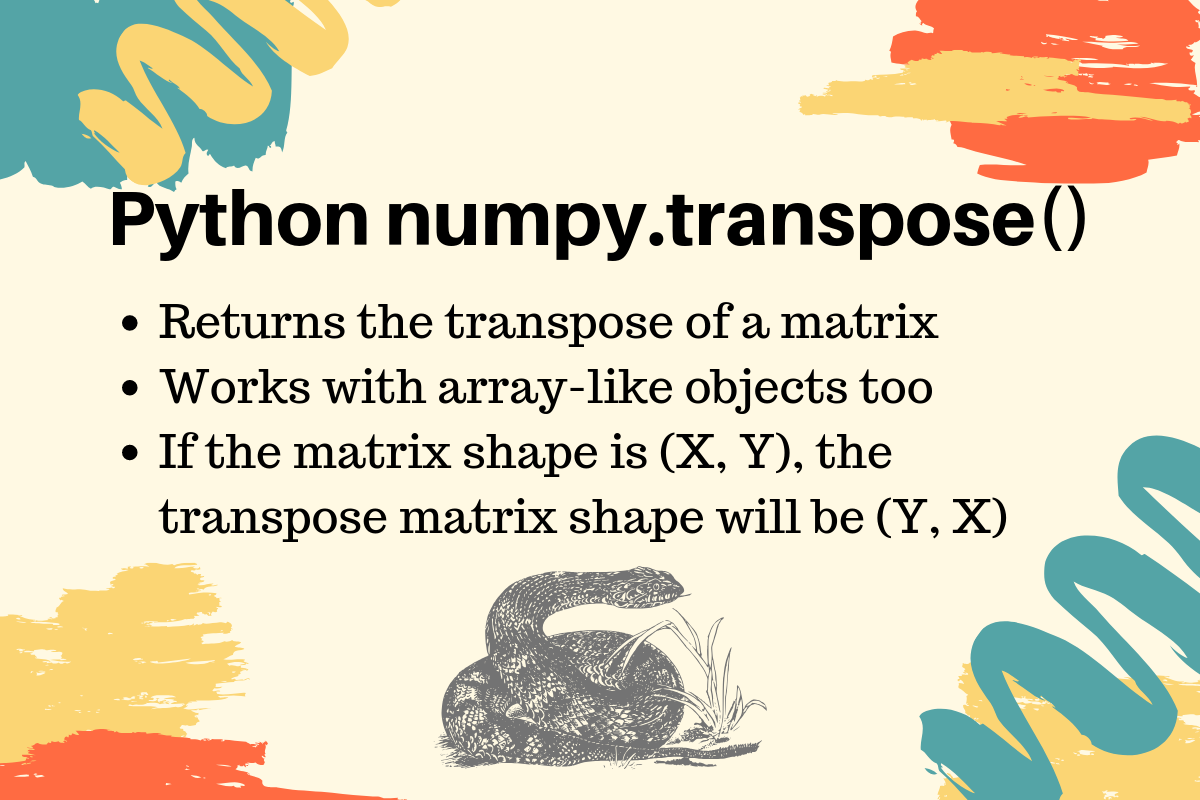# NumPy Matrix transpose() - Transpose of an Array in Python

Published on August 3, 2022By Pankaj
Developer and author at DigitalOcean.While we believe that this content benefits our community, we have not yet thoroughly reviewed it. If you have any suggestions for improvements, please let us know by clicking the “report an issue“ button at the bottom of the tutorial.

The transpose of a matrix is obtained by moving the rows data to the column and columns data to the rows. If we have an array of shape (X, Y) then the transpose of the array will have the shape (Y, X).

## NumPy Matrix transpose()

Python numpy module is mostly used to work with arrays in Python. We can use the transpose() function to get the transpose of an array.

``````import numpy as np

arr1 = np.array([[1, 2, 3], [4, 5, 6]])

print(f'Original Array:\n{arr1}')

arr1_transpose = arr1.transpose()

print(f'Transposed Array:\n{arr1_transpose}')
``````

Output:

``````Original Array:
[[1 2 3]
[4 5 6]]
Transposed Array:
[[1 4]
[2 5]
[3 6]]
``````

## Transpose of an Array Like Object

The transpose() function works with an array like object too, such as a nested list.

``````arr1 = [[1, 2, 3], [4, 5, 6]]

arr1_transpose = np.transpose(arr1)
``````

The result will be the same as the earlier program. Reference: API DocPankaj

author

Developer and author at DigitalOcean.

#### Still looking for an answer?

Can you please explain that how can I pass 2d np array from python to c ?

- Amrit

Great Article.Can you elaborate on the axes attribute of transpose

- Vedant